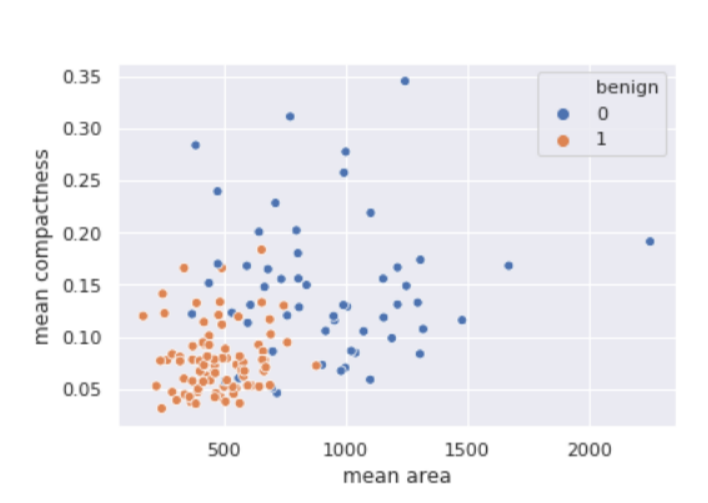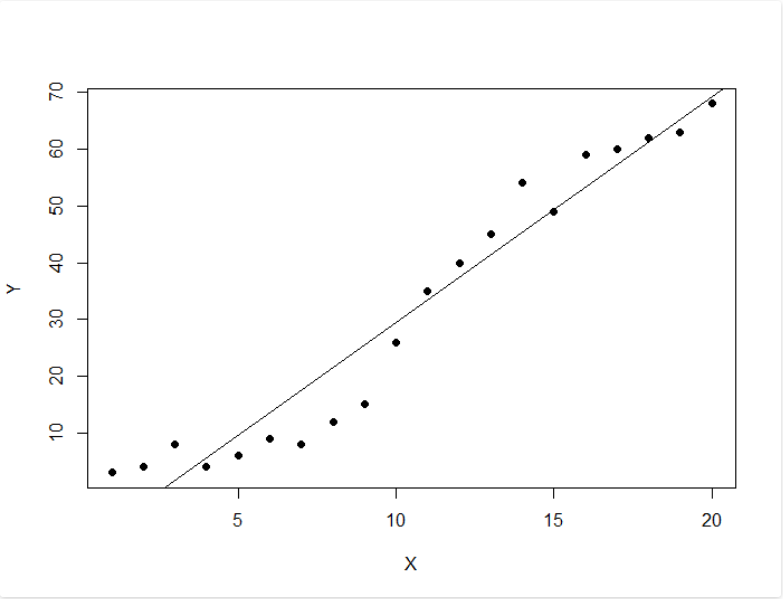# Classification in R Programming

R is a very dynamic and versatile programming language for data science. This article deals with classification in R. Generally classifiers in R are used to predict specific category related information like reviews or ratings such as good, best or worst.

Various Classifiers are:

• Decision Trees
• Naive Bayes Classifiers
• K-NN Classifiers
• Support Vector Machines(SVM’s)

#### Decision Tree Classifier

It is basically is a graph to represent choices. The nodes or vertices in the graph represent an event and the edges of the graph represent the decision conditions. Its common use is in Machine Learning and Data Mining applications.

Applications:
Spam/Non-spam classification of email, predicting of a tumor is cancerous or not. Usually, a model is constructed with noted data also called training dataset. Then a set of validation data is used to verify and improve the model. R has packages that are used to create and visualize decision trees.

The R package “party” is used to create decision trees.
Command:

`install.packages("party")`

 `# Load the party package. It will automatically load other ` `# dependent packages. ` `library(party) ` ` `  `# Create the input data frame. ` `input``.dat <``-` `readingSkills[c(``1``:``105``), ] ` ` `  `# Give the chart file a name. ` `png(``file` `=` `"decision_tree.png"``) ` ` `  `# Create the tree. ` `  ``output.tree <``-` `ctree( ` `  ``nativeSpeaker ~ age ``+` `shoeSize ``+` `score,  ` `  ``data ``=` `input``.dat) ` ` `  `# Plot the tree. ` `plot(output.tree) ` ` `  `# Save the file. ` `dev.off() `

Output:

```null device
1

Attaching package: ‘zoo’

The following objects are masked from ‘package:base’:

as.Date, as.Date.numeric

#### Naive Bayes Classifier

Naïve Bayes classification is a general classification method that uses a probability approach, hence also known as a probabilistic approach based on Bayes’ theorem with the assumption of independence between features. The model is trained on training dataset to make predictions by `predict()` function.

Formula:

`P(A|B)=P(B|A)×P(A)P(B)`

It is a sample method in machine learning methods but can be useful in some instances. The training is easy and fast that just requires considering each predictor in each class separately.

Application:
It is used generally in sentimental analysis.

 `library(caret) ` `## Warning: package 'caret' was built under R version 3.4.3 ` `set``.seed(``7267166``) ` `trainIndex ``=` `createDataPartition(mydata\$prog, p ``=` `0.7``)\$Resample1 ` `train ``=` `mydata[trainIndex, ] ` `test ``=` `mydata[``-``trainIndex, ] ` ` `  `## check the balance ` `print``(table(mydata\$prog)) ` `##  ` `## academic    general vocational  ` `## 105         45         50 ` `print``(table(train\$prog)) `

Output:

```## Naive Bayes Classifier for Discrete Predictors
##
## Call:
## naiveBayes.default(x = X, y = Y, laplace = laplace)
##
## A-priori probabilities:
## Y
##  0.5248227  0.2269504  0.2482270
##
## Conditional probabilities:
##             science
## Y                [, 1]     [, 2]
##   general    52.18750 8.847954
##   vocational 47.31429 9.969871
##
##             socst
## Y                [, 1]      [, 2]
##   general    51.12500  8.377196
##   vocational 44.82857 10.279865```

#### K-NN Classifier

Another used classifier is the K-NN classifier. In pattern recognition, the k-nearest neighbor’s algorithm (k-NN) is a non-parametric method generally used for classification and regression. In both cases, the input consists of the k closest training examples in the feature space. In k-NN classification, the output is a class membership.
Applications:
Used in a variety of applications such as economic forecasting, data compression, and genetics.

Example:

 `# Write Python3 code here ` `import` `numpy as np ` `import` `pandas as pd ` `from` `matplotlib ``import` `pyplot as plt ` `from` `sklearn.datasets ``import` `load_breast_cancer ` `from` `sklearn.metrics ``import` `confusion_matrix ` `from` `sklearn.neighbors ``import` `KNeighborsClassifier ` `from` `sklearn.model_selection ``import` `train_test_split ` `import` `seaborn as sns ` `sns.``set``() ` `breast_cancer ``=` `load_breast_cancer() ` `X ``=` `pd.DataFrame(breast_cancer.data, columns ``=` `breast_cancer.feature_names) ` `X ``=` `X[[``'mean area'``, ``'mean compactness'``]] ` `y ``=` `pd.Categorical.from_codes(breast_cancer.target, breast_cancer.target_names) ` `y ``=` `pd.get_dummies(y, drop_first ``=` `True``) ` `X_train, X_test, y_train, y_test ``=` `train_test_split(X, y, random_state ``=` `1``) ` `sns.scatterplot( ` `    ``x ``=``'mean area'``, ` `    ``y ``=``'mean compactness'``, ` `    ``hue ``=``'benign'``, ` `    ``data ``=` `X_test.join(y_test, how ``=``'outer'``) ` `) `

Output:#### Support Vector Machines(SVM’s)

A support vector machine (SVM) is a supervised binary machine learning algorithm that uses classification algorithms for two-group classification problems. After giving an SVM model sets of labeled training data for each category, they’re able to categorize new text.

Mainly SVM is used for text classification problems. It classifies the unseen data. It is widely used than Naive Bayes.SVM id usually a fast and dependable classification algorithm that performs very well with a limited amount of data.

Applications:
SVMs have a number of applications in several fields like Bioinformatics, to classify genes, etc.

Example:

 `# Load the data from the csv file ` `dataDirectory <``-` `"D:/"` `# put your own folder here ` `data <``-` `read.csv(paste(dataDirectory, ``'regression.csv'``, sep ``=``""), header ``=` `TRUE) ` ` `  `# Plot the data ` `plot(data, pch ``=` `16``) ` ` `  `# Create a linear regression model ` `model <``-` `lm(Y ~ X, data) ` ` `  `# Add the fitted line ` `abline(model) `

Output:My Personal Notes arrow_drop_upCheck out this Author's contributed articles.

If you like GeeksforGeeks and would like to contribute, you can also write an article using contribute.geeksforgeeks.org or mail your article to contribute@geeksforgeeks.org. See your article appearing on the GeeksforGeeks main page and help other Geeks.

Please Improve this article if you find anything incorrect by clicking on the "Improve Article" button below.

Article Tags :

Be the First to upvote.

Please write to us at contribute@geeksforgeeks.org to report any issue with the above content.